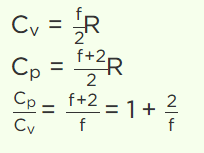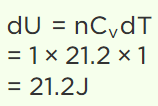## Kinetic Theory Questions and Answers Part-17

1. A cylinder rolls without slipping down an inclined plane, the number of degrees of freedom it has, is
a) 2
b) 3
c) 5
d) 1

Explanation: A cylinder rolls without slipping down an inclined plane, the number of degrees of freedom it has, is 2

2. If the degree of freedom of a gas are f, then the ratio of two specific heats $C_{P}\diagup C_{V}$  is given by
a) $\frac{2}{f}+1$
b) $1-\frac{2}{f}$
c) $1+\frac{1}{f}$
d) $1-\frac{1}{f}$

Explanation:3. The degrees of freedom of a triatomic gas is
a) 2
b) 4
c) 6
d) 8

Explanation: The degrees of freedom of a triatomic gas is 6

4. A diatomic gas molecule has translational, rotational and vibrational degrees of freedom. The $C_{P}\diagup C_{V}$  is
a) 1.67
b) 1.4
c) 1.29
d) 1.33

Explanation: A diatomic gas molecule has translational, rotational and vibrational degrees of freedom. The $C_{P}\diagup C_{V}$  is 1.33

5. For a gas $\frac{R}{C_{V}}=0.67$   . This gas is made up of molecules which are
a) Diatomic
b) Mixture of diatomic and polyatomic molecules
c) Monoatomic
d) Polyatomic

Explanation: For a gas $\frac{R}{C_{V}}=0.67$   . This gas is made up of molecules which are Monoatomic

6. The value of CV for one mole of neon gas is
a) $\frac{1}{2}R$
b) $\frac{3}{2}R$
c) $\frac{5}{2}R$
d) $\frac{7}{2}R$

Explanation: The value of CV for one mole of neon gas is $\frac{3}{2}R$

7. For an ideal gas of diatomic molecules
a) $C_{P}=\frac{5}{2}R$
b) $C_{V}=\frac{3}{2}R$
c) $C_{P}-C_{V}=2R$
d) $C_{P}=\frac{7}{2}R$

Explanation: For an ideal gas of diatomic molecules, $C_{P}=\frac{7}{2}R$

8. At constant volume, for different diatomic gases the molar specific heat is
a) Same and 3 cal/mole/°C approximately
b) Exactly equal and its value is 4 cal/mole/°C
c) Will be totally different
d) Approximately equal and its value is 5 cal/mole/°C

Explanation: At constant volume, for different diatomic gases the molar specific heat is approximately equal and its value is 5 cal/mole/°C

9. At constant volume the specific heat of a gas is $\frac{3R}{2}$ , then the value of '$\gamma$ ' will be
a) $\frac{3}{2}$
b) $\frac{5}{2}$
c) $\frac{5}{3}$
d) None of the above

Explanation: $\frac{5}{3}$